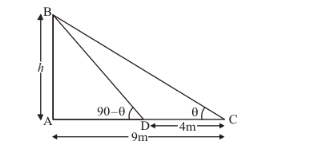# The angles of elevation of the top of a tower from two points

Question:

The angles of elevation of the top of a tower from two points at a distance of 4 m and 9 m from the base of the tower and in the same straight line with it are complementary. Prove that the height of the tower is 6m.

Solution:

Letbe tower of heightm and angle of elevation of the top of tower from two points areandLet,m andm andThe corresponding figure is as followsSo we use trigonometric ratios.

In,

$\Rightarrow \tan \theta=\frac{A B}{A C}$

$\Rightarrow \tan \theta=\frac{h}{4}$

Again in $\triangle A B D$,

$\Rightarrow \tan (90-\theta)=\frac{A B}{A D}$

$\Rightarrow \quad \tan \theta=\frac{9}{h}$

$\Rightarrow \quad \frac{h}{4}=\frac{9}{h}$

$\Rightarrow \quad h=6$

Hence the height of tower is $6 \mathrm{~m}$.